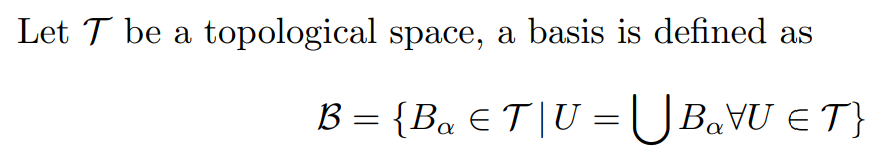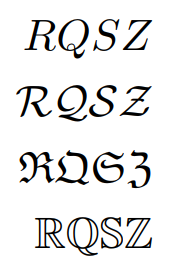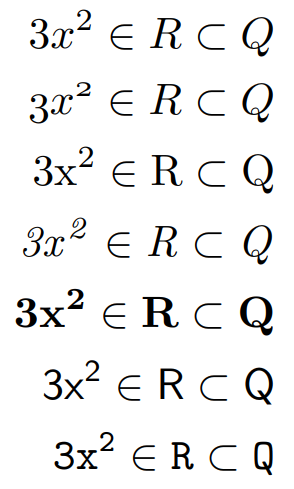Introduction

Some mathematical elements need to be typeset using fonts containing characters/symbols of a certain style; for example, it is customary to represent real numbers with a blackboard bold font (such as $$\mathbb{R}$$), or topological spaces with calligraphic font (such as ($$\mathcal{T}$$). This article shows how to use different font styles when typesetting mathematics, starting with the following example:

Let $$\mathcal{T}$$ be a topological space, a basis is defined as
$\mathcal{B} = \{B_{\alpha} \in \mathcal{T}\, |\, U = \bigcup B_{\alpha} \forall U \in \mathcal{T} \}$

This example produces the following output:Use of some math font styles requires the line \usepackage{amssymb} to be added to the document preamble: see the amsfonts package for further information.

Capital letters-only font typefaces

There are some font typefaces which support only a limited number of characters; these fonts usually denote some special sets. For instance, to display the R in blackboard bold typeface you can use $$\mathbb{R}$$ to produce $$\mathbb{R}$$. The following example shows calligraphic, fraktur and blackboard bold typefaces:

\documentclass{article}
\usepackage{amsmath}
\usepackage{amssymb}
\begin{document}
\begin{align*}
RQSZ \\
\mathcal{RQSZ} \\
\mathfrak{RQSZ} \\
\mathbb{RQSZ}
\end{align*}
\end{document}

This example produces the following output:Other mathematical fonts

It is possible to set a different font family for a complete mathematical expression:

\begin{align*}
3x^2 \in R \subset Q \\
\mathnormal{3x^2 \in R \subset Q} \\
\mathrm{3x^2 \in R \subset Q} \\
\mathit{3x^2 \in R \subset Q} \\
\mathbf{3x^2 \in R \subset Q} \\
\mathsf{3x^2 \in R \subset Q} \\
\mathtt{3x^2 \in R \subset Q}
\end{align*}

This example produces the following output:In this case, not only letters but all characters change their appearance; for example, $\mathit{3x^2}$ italicises the entire expression to produce $$\mathit{3x^2}$$.